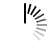# Octave Programming Examples

## Average values using Octave

```% set matrix
Patient_HR = [ 40 45 50 47; 68 66 68 69; 77 75 76 78 ]

% get matrix row and height
[ rows, columns ] = size(Patient_HR)

% create a n-row, single column matrix of zeros
AverageHR = zeros( rows, 1 )

% loop through each row in matrix
for index = 1 : rows
% assign temp to row
temp = Patient_HR(index, :)

% average values in temp row and set in AverageHR matrix
AverageHR(index, 1) = mean(temp)
end```

## you-cheated-on-your-homework-problem-5

```Patient_HR = [ 40 45 50 47; 68 66 68 69; 77 75 76 78 ]
AverageHR = [ 0; 0; 0 ]

[ rows, columns ] = size(Patient_HR)

for index = 1 : rows
temp = Patient_HR(index, :)
AverageHR(index, 1) = mean(temp)
end```

## ejercicioenclase

```suma = 0;
y=0;
h=0;
x=0.3*pi;
total = 0;
vv=0;
for n = 0:2:60
(n/2)+1
suma = (((-1)^y*(x^n)/(factorial(n))))
total = total + suma
vv = cos(suma)
y=y+1
end
%Ejercicio hecho en clase 10/04/2019```

## hola

```x=0.5;
respuesta=0;
total=0;
for i=0:1:60
respuesta=((x^[i]))/(factorial(i));
total=respuesta+total
end
total```

## plot

```function[R,LEL]=plot(hm,hb,mhz)
R=10;
hm=6.7;
hb=46.1;
mhz=461.67;
oc=69.55+26.16*(log10(mhz));
ahm=3.2*((log10(11.75*(hm)))^2)-1.1;
A=oc-13.82*(log10(hb))-ahm;
B=44.9-6.55*(log10(hb));
LEL=A+B*log10(R);
end

```

## Execute MATLAB/Octave Online

```exts = {'.jpg','.png'};
fileNames = {'sherlock.jpg','car2.jpg','fabric.png','greens.jpg','hands1.jpg','kobi.png',...
'lighthouse.png','micromarket.jpg','office_4.jpg','onion.png','pears.png','yellowlily.jpg',...
'indiancorn.jpg','flamingos.jpg','sevilla.jpg','llama.jpg','parkavenue.jpg',...
'peacock.jpg','car1.jpg','strawberries.jpg','wagon.jpg'};
filePath = [fullfile(matlabroot,'toolbox','images','imdata') filesep];
filePathNames = strcat(filePath,fileNames);
testImages = datastore(filePathNames,'FileExtensions',exts);```

## ImposeZeroesPoly

```x = [1 2 3 4 5 6 7 8 9 10];
y1 = [.16 .08 .04 .02 .013 .007 .004 .002 .001 .0008 ];
y2 = [.16 .07 .03 .01 .008 .003 .0008 .0003 .00007 .00002 ];

semilogy(x,y1,'-bo;y1;',x,y2,'-kx;y2;');
title('Plot title');
xlabel('X Axis');
ylabel('Y Axis');

print -dpng figure.png

```

## Execute MATLAB/Octave Online

```clear, clc, close all

[x, fs] = wavread('C:\Users\student\Downloads\sample.wav');    % get the sound file
x = x(:, 1);                        % get the first channel
xmax = max(abs(x));                 % find the maximum value
x = x/xmax;                         % scale the signal

% time vector generation
N = length(x);
t = (0:N-1)/fs;

% cepstral analysis
[C, q] = cepstrum(x, fs);

% plot of the signal
figure(1)
subplot(2, 1, 1)
plot(t, x, 'r')
xlim([0 max(t)])
ylim([-1.1*max(abs(x)) 1.1*max(abs(x))])
grid on
set(gca, 'FontName', 'Times New Roman', 'FontSize', 14)
xlabel('Time, s')
ylabel('Normalized amplitude')
title('The signal in the time domain')

% plot of the cepstrum
% 1 ms minimum speech quefrency (1000 Hz) and 20 ms maximum speech quefrency (50 Hz)
subplot(2, 1, 2)
plot(q*1000, C, 'r');
grid on
xlim([1 20])
set(gca, 'FontName', 'Times New Roman', 'FontSize', 14)
xlabel('Quefrency, ms')
ylabel('Amplitude')
title('Amplitude cepstrum of the signal (quefrencies from 1 ms to 20 ms)')```

## Execute MATLAB/Octave Online

```doc randi

```

## YEUNGKAWING20353855_4

```function PowerIter

n=500;
A=randn(n,n);
A=(A+A')/2;

for testtime=1:5
y0=randn(n,1);
y0=y0/norm(y0);
[mu,y]=Power(A,y0);
% Store mu and y
mu1(testtime)=mu;
y1(:,testtime)=y;
end
% Display the results
disp('The computed eigenvalues are shown below. You will see they are almost the same.')
disp(mu1)
disp('The inner products of computed eigenvectors are shown below.');
disp('You will see some are close to 1, and some are close to -1.');
disp('This shows that the computed vectors may be in opposite direction');
disp('but both are the same eigenvector.')
disp(y1'*y1)

% Below is the subroutine of power iteration.
function [mu,y]=Power(A,y0)
maxit=10^4;
epsilon=1e-6;
y=y0;
for iter=1:maxit
%%%%%%%
%Insert your lines for Power Iteration
m^(k)=A*y^(k-1)
y^(k)=m^(k)/abs (m^k)_2
U^(k)=(y^(k))'*A*(y^(k))

%%%%%%%
% Check: if ||Ay^k - mu^k y^k ||_2<= epsilon, then break the
% for loop.
% Complete the following line
if
abs (A*y^k - m*u^k y^k)_2 <= epsilon
break;
end
end

end

end
```

AdvertisementsWe use cookies to provide and improve our services. By using our site, you consent to our Cookies Policy.